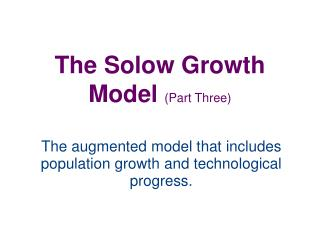DownloadDownload PresentationThe Solow Growth Model (Part Three)

# The Solow Growth Model (Part Three)

Télécharger la présentation## The Solow Growth Model (Part Three)

- - - - - - - - - - - - - - - - - - - - - - - - - - - E N D - - - - - - - - - - - - - - - - - - - - - - - - - - -
##### Presentation Transcript

1. The Solow Growth Model (Part Three) The augmented model that includes population growth and technological progress.

2. Model Background • As mentioned in parts I and II, the Solow growth model allows us a dynamic view of how savings affects the economy over time. We learned about the steady state level of capital and how a golden rule steady state level of capital can be achieved by setting the savings rate to maximize consumption per worker. We now augment the model to see the effects of population growth and technological progress.

3. Steady State Equilibrium • By expanding our model to include population growth our model more closely resembles the sustained economic growth observable in much of the real world. • To see how population growth affects the steady state we need to know how it affects the accumulation of capital per worker. When we add population growth (n) to our model the change in capital stock per worker becomes…Δk = i – (δ+n)k • As we can see population growth will have a negative effect on capital stock accumulation. We can think of (δ+n)k as break-even investment or the amount of investment necessary to keep capital stock per worker constant. • Our analysis proceeds as in the previous presentations. To see the impact of investment, depreciation, and population growth on capital we use the (change in capital) formula from above,Δk = i – (δ+n)k …substituting for (i) gives us,Δk = s*f(k) – (δ+n)k

4. Steady State Equilibrium with population growth Break-even investment(δ+n)k s*f(k)Investment • At the point where both (k) and (y) are constant it must be the case that,Δk = s*f(k) – (δ+n)k = 0 …or,s*f(k) = (δ+n)k…this occurs at our equilibrium point k*. Like depreciation, population growth is one reason why the capital stock per worker shrinks. InvestmentBreak-even Investment s*f(k*)=(δ+n)k* k k* At k* break-even investment equals investment.

5. The impact of population growth • Suppose population growth changes from n1 to n2. • This shifts the line representing population growth and depreciation upward. An increase in “n” InvestmentBreak-even Investment (δ+n2)k (δ+n1)k • At the new steady state k2* capital per worker and output per worker are lower • The model predicts that economies with higher rates of population growth will have lower levels of capital per worker and lower levels of income. s*f(k) k k2* k1* …reduces k*

6. The efficiency of labour • We rewrite our production function as…Y=F(K,L*E)where “E” is the efficiency of labour. “L*E” is a measure of the number of effective workers. The growth of labour efficiency is “g”. • Our production function y=f(k) becomes output per effective worker since…y=Y/(L*E) and k=K/(L*E) • With this augmentation “δk” is needed to replace depreciating capital, “nk” is needed to provide capital to new workers, and “gk” is needed to provide capital for the new effective workers created by technological progress.

7. Steady State Equilibrium with population growth and technological progress • At the point where both (k) and (y) are constant it must be the case that,Δk = s*f(k) – (δ+n+g)k = 0 …or,s*f(k) = (δ+n)k…this occurs at our equilibrium point k*. Like depreciation and population growth, the labour augmenting technological progress rate causes the capital stock per worker to shrink. Break-even investment(δ+n+g)k InvestmentBreak-even Investment s*f(k)Investment s*f(k*)=(δ+n)k* At k* break-even investment equals investment. k k*

8. The impact of technological progress • Suppose the worker efficiency growth rate changes from g1 to g2. • This shifts the line representing population growth, depreciation, and worker efficiency growth upward. An increase in “g” InvestmentBreak-even Investment (δ+n+g2)k (δ+n+g1)k s*f(k) • At the new steady state k2* capital per worker and output per worker are lower. • The model predicts that economies with higher rates of worker efficiency growth will have lower levels of capital per worker and lower levels of income. k k2* k1* …reduces k*

9. Effects of technological progress on the golden rule • With technological progress the golden rule level of capital is defined as the steady state that maximizes consumption per effective worker. Following our previous analysis steady state consumption per worker is…c* = f(k*) – (δ+ n + g)k* • To maximize this…MPK = δ+ n + gorMPK – δ= n + g • That is, at the Golden Rule level of capital, the net marginal product of capital MPK – δ, equals the rate of growth of total output, n+g.

10. Steady State Growth Rates in the Solow Model with Technological Progress

11. Conclusion • In this section we added changes in two exogenous variables (population and technological growth) to the Solow growth model. We saw that in steady state output per effective worker remains constant, output per worker depends only on technological growth, and that Total output depends on population and technological growth.